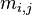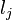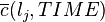Algorithm for generalized net functioning

Below we will describe the most general algorithm for the generalized net functioning.

For this purpose, we will introduce the concept of Abstract Transition (AT). This is a GN transition represented as the union of all active GN-transitions at a given time-moment. For its construction the operation "union" of transitions is used (see Operations and relations over generalized nets).

The algorithm

 Step B01 Put all tokens α, for which θK(α) ≤ T, into the corresponding input places of the net. Step B02 Construct the GN's Abstract Transition (initially it is empty). Step B03 Check whether the value of the current time is less than T + t*. Step B04 If the answer to the question on Step B03 is "no", terminate the process of GN functioning. Step B05 Check all transitions for which the first time-component is exactly equal to the current time-moment. Step B06 Check the transition's types of all transitions determined by B05. The method of checking is as follows: Change the names of all places which participate in the Boolean expression of the transition type as variables with values: 0, if the corresponding place has no tokens at the current moment; 1, otherwise; Calculate the truth value of the so obtained Boolean expression. Step B07 Add all transitions from Step B06, for which the transition types are satisfied by the Abstract Transition. Step B08 Apply the algorithm for transition functioning over the Abstract Transition. Step B09 Remove from the Abstract Transition all transitions, which are inactive at the current time-moment. Step B10 Increase the current time with t0. Step B11 Go to Step B03.

This algorithm helps us to realize the different subprocesses (which exist in the frames of the most global modelled process) on the level of places, while analogous processes in other nets are modelled on the level of transitions. Moreover, the places are sorted by their priorities while in other nets this is not the case. Therefore, the sequence of the process realization by generalized nets is performed on the level of places (from different active transitions). Furthermore, when some places have equal priority, the tokens' transfer is related to the tokens' priorities, which is difficult to achieve in other nets.

This algorithm was described in the "Generalized Nets" from 1991 year  and "On Generalized Nets Theory" from 2007 year .

Conclusions

The algorithm guarantees that no conflict situations may occur during the functioning of the generalized net, due to the preliminary prioritization of the transitions and the places, and the predicates.

However, a condition may be formulated for which a given GN will not have the possibility to continue functioning, but will have to wait. This condition is represented as a double sum of products.

The process of GN functioning will temporarily stop, if$\sum_{i=1}^m \sum_{j=1}^n f(r_{i,j}) . m_{i,j}(TIME) . \overline{c}(l_i, TIME) . (c(l_j) - \overline{c}(l_j, TIME)) = 0$

where:

•$f(r_{i,j})= \begin{cases}1 \text{ if the predicate } r_{i,j} \text{ is true'' } \\ 0 \text{ otherwise } \end{cases}$
•$m_{i,j}$ is the capacity of this arc, which decrements with 1 for each token that passes during the transfer at the current moment$TIME$. When it reaches zero, the arc capacity will be fully utilized and no more tokens may pass at this time.
•$\overline{c}(l_i, TIME)$ is the number of tokens, which at moment TIME stay in the input place$l_i$. When there are no tokens in the input place, no transfer may occur and the process will stop.
•$(c(l_j) - \overline{c}(l_j, TIME))$ is the number of vacancies in the output place$l_j$ at current moment$TIME$, formed as a difference between the standard maximal number of tokens$c(l_j)$ and the current number of tokens there$\overline{c}(l_j, TIME)$. If there are no vacancies in the output place, no tokens from the input place may transfer.